Question

# write state-space model matrices and obtain the transfer function H(s)=Y(s)U(s) (please use the matlab simulink)

write state-space model matrices and obtain the transfer function H(s)=Y(s)U(s)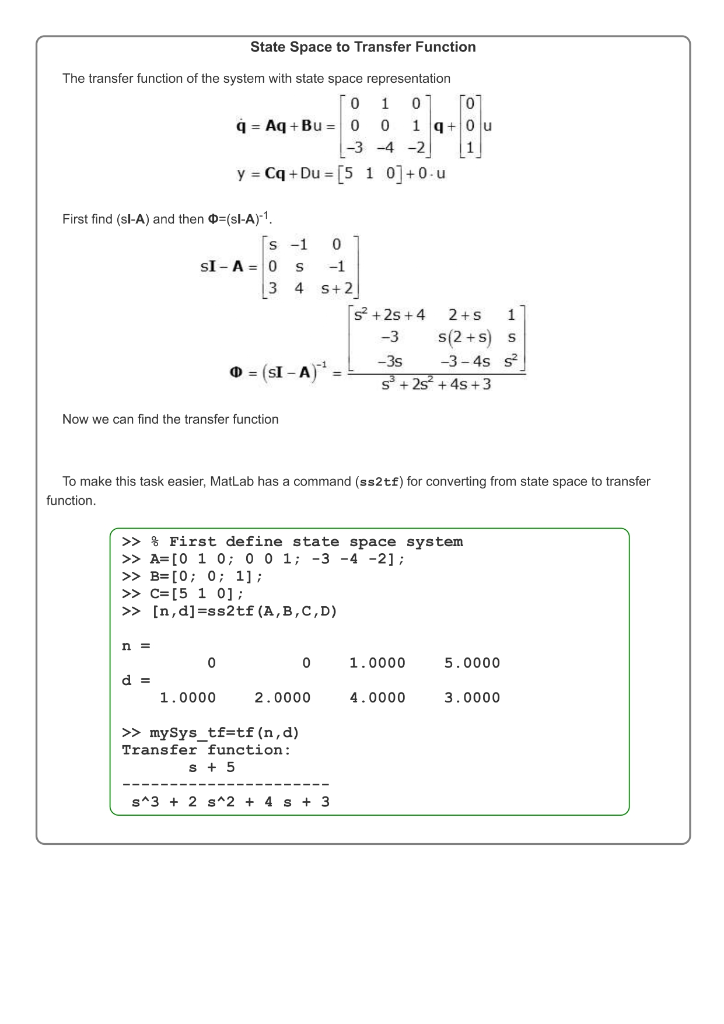Please upvote our work. THANK YOU !!

#### Earn Coins

Coins can be redeemed for fabulous gifts.

Similar Homework Help Questions
• ### ! Required information Use MATLAB to obtain a state variable model. Consider the following transfer function:...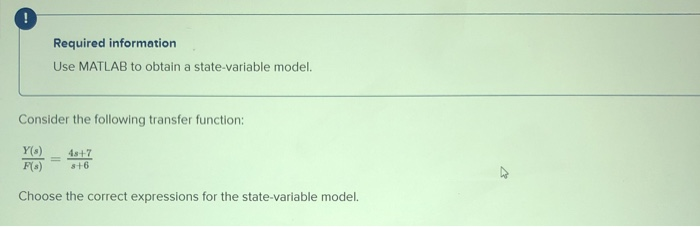! Required information Use MATLAB to obtain a state variable model. Consider the following transfer function: 4s+7 Y(s) F() Choose the correct expressions for the state variable model. Multiple Choice ¿= -3x + 2f and y= -6x +4f = -6x +4f and y= -4.25x +4f 3 = 6x-4f and y-4.25- 4f O i = 3x – 2f and y = 62 – 4f

• ### from the following model of a discrete process in the state space obtain its transfer function...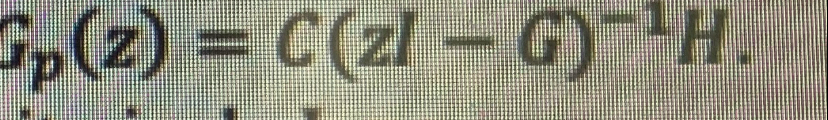from the following model of a discrete process in the state space obtain its transfer function given by fp(z) = C(ZI – G)-'H. x(k+1) = 11.23 1.63] «(k) +  u(k) y(k) = [1 0]x(k)

• ### Required information Use MATLAB to create a state-variable model and obtain the expressions for the matrices...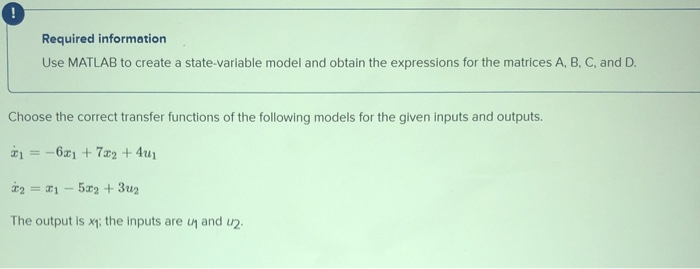Required information Use MATLAB to create a state-variable model and obtain the expressions for the matrices A, B, C, and D. Choose the correct transfer functions of the following models for the given inputs and outputs. -6a1 + 782 + 4u 2 = x1 - 522 + 3u2 The output is xy: the inputs are u and u2. Multiple Choice X () U.) 4s+20 and 2-11-23 X,() Us(s) 21 2-118-23 Ui() 45-+20 2+11s+23 and X U (0) 21 2+115+23 X;)...

• ### ! Required information Use MATLAB to obtain a state variable model. Consider the following transfer function:...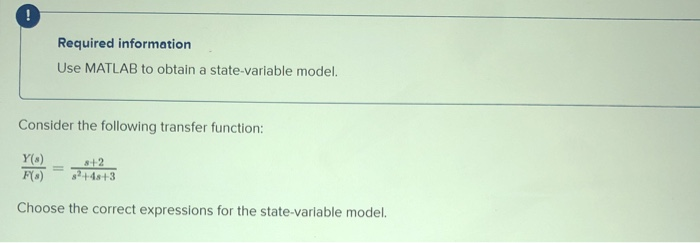! Required information Use MATLAB to obtain a state variable model. Consider the following transfer function: Y(3) F(*) 8+2 3+48+3 Choose the correct expressions for the state-variable model. Multiple Choice 1 = -4c1 - 1.522 +2f, *2 =221, and y=0.581 +0.5x2 1 -41 -202 +2f, 2 = 2.01, and y=2.01 -0.5x2 1 = -2x1 - 3x2 +2f, -81, and y21-22 1 - - 2x1 - 362 +28,62 -4.01, and y2 +0.5x2

• ### Consider a single input, single output system with transfer function 10 H(s)- s+10s +25s +100 Obtain a state-space...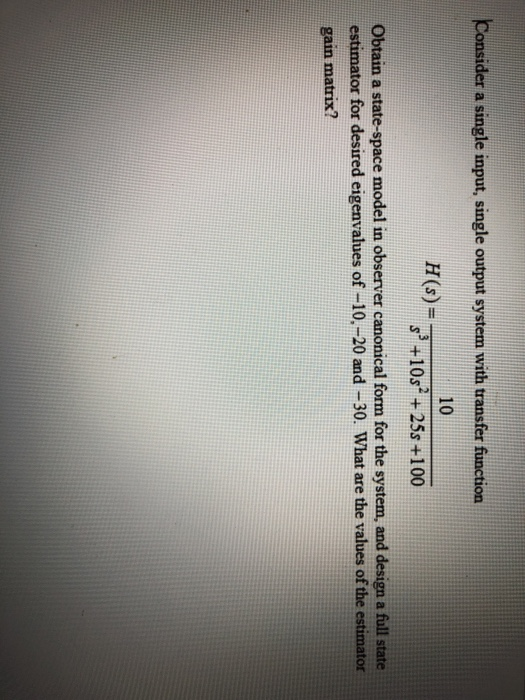Consider a single input, single output system with transfer function 10 H(s)- s+10s +25s +100 Obtain a state-space model in observer canonical form for the system, and design a full state estimator for desired eigenvalues of -10.-20 and-30. What are the values of the estimator gain matrix? Consider a single input, single output system with transfer function 10 H(s)- s+10s +25s +100 Obtain a state-space model in observer canonical form for the system, and design a full state estimator for...

• ### can you show how the model would look like on matlab ? please help with this question For the circuit given below, find the normal form for the inductor current and capacitor voltage. Use Matlab (...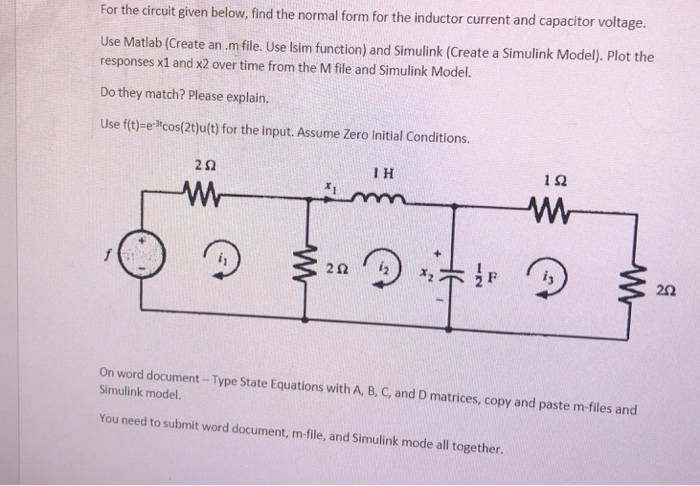can you show how the model would look like on matlab ? please help with this question For the circuit given below, find the normal form for the inductor current and capacitor voltage. Use Matlab (Create an.m file. Use Isim function) and Simulink (Create a Simulink Model). Plot the responses x1 and x2 over time from the M file and Simulink Model. Do they match? Please explain. Use f(t)-e-cos(2t)u(t) for the input. Assume Zero Initial Conditions. 2Ω I H On...

• ### can you show how the model would look like on matlab ? please help with this...can you show how the model would look like on matlab ? please help with this question For the circuit given below, find the normal form for the inductor current and capacitor voltage. Use Matlab (Create an.m file. Use Isim function) and Simulink (Create a Simulink Model). Plot the responses x1 and x2 over time from the M file and Simulink Model. Do they match? Please explain. Use f(t)-e-cos(2t)u(t) for the input. Assume Zero Initial Conditions. 2Ω I H On...

• ### ! Required information Use MATLAB to create a state-variable model and obtain the expressions for the...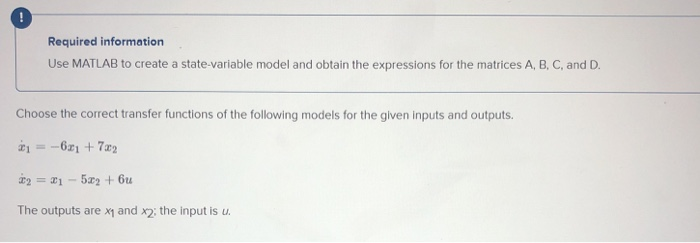! Required information Use MATLAB to create a state-variable model and obtain the expressions for the matrices A, B, C, and D. Choose the correct transfer functions of the following models for the given inputs and outputs. -621 + 7x2 2 = 21 - 5x2 + bu The outputs are xy and x2; the input is u. Multiple Choice X.) UG) 42 2+11+23 and X(s) U() 6s+36 2+115+23 X) U) 36 32+10s+23 and U) 68+42 +105+23 25 32-10-18 and U(3)...

• ### Use matlab to obtain a state-space representation of C(s) G(s)= E(s) 25° +4.88² +9.65 +16 S+5...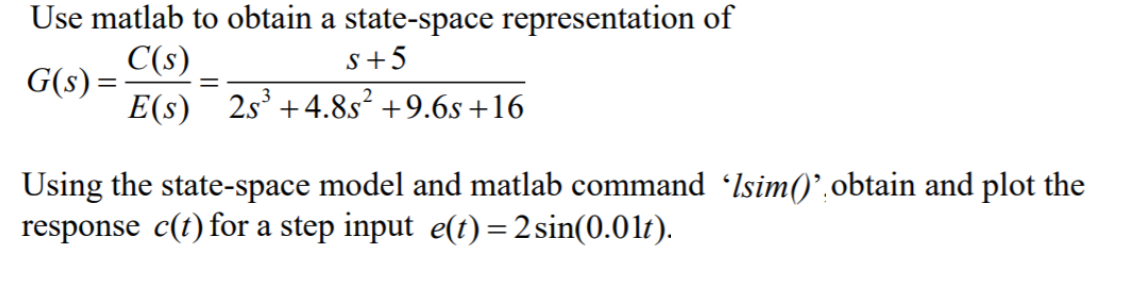Use matlab to obtain a state-space representation of C(s) G(s)= E(s) 25° +4.88² +9.65 +16 S+5 Using the state-space model and matlab command ‘Isim()”.obtain and plot the response c(t) for a step input e(t) = 2 sin(0.01t).

• ### Required information Use MATLAB to create a state variable model and obtain the expressions for the...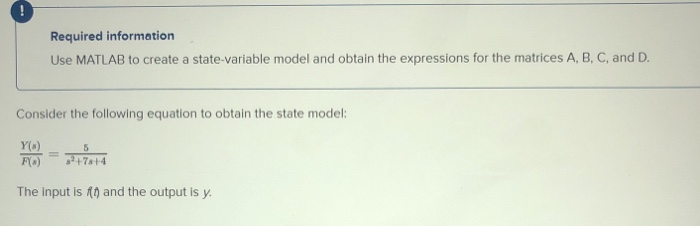Required information Use MATLAB to create a state variable model and obtain the expressions for the matrices A, B, C, and D. Consider the following equation to obtain the state model: Y() F(x) The input is no) and the output is y. Multiple Choice A = [0 1.25], B- - , 0 - 1 - 2 - 3 and D -(0) O A-173],3-[a] .c -60, and D - 10 1.25) A-[7.], B-[].C-10 1.25), and D - (0) A-10), B =[],c-...